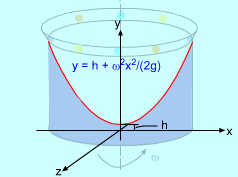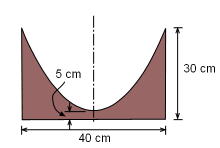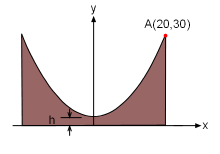Ch 9. Integrate Application Multimedia Engineering Math Area BetweenCurves Volume Work Average Value
 Chapter 1. Limits 2. Derivatives I 3. Derivatives II 4. Mean Value 5. Curve Sketching 6. Integrals 7. Inverse Functions 8. Integration Tech. 9. Integrate App. 10. Parametric Eqs. 11. Polar Coord. 12. Series Appendix Basic Math Units Search eBooks Dynamics Fluids Math Mechanics Statics Thermodynamics Author(s): Hengzhong Wen Chean Chin Ngo Meirong Huang Kurt Gramoll ©Kurt GramollMATHEMATICS - CASE STUDY IntroductionMold Shape In his pottery class, Roy noticed that when cylindrical container with clay is rotated, the clay forms a parabolic shape. After some experimenting, he notices that the surface of the clay is a paraboloid of revolution generated by rotating the parabola  y = h + ω2 x2/(2g) about the y-axis, where ω is the angular velocity and g is the gravitational acceleration. He now wonders how he can determine the angular velocity so that the clay will conform to a given height and width. He would like to use this for a mold. What is known: Mold size required is 30 cm high and 40 cm wide as shown in the diagram at the left.Magnitude of the Mold Questions What is the angular velocity required so the clay is 30 cm high at the outside edge and 5 cm high at the center? Also, what is the total volume of clay needed?Coordinate System Approach Use the point A(20, 30) to help determining the angular velocity. This is a cylindrical shell (see theory). Use the coordinate system shown in the diagram.